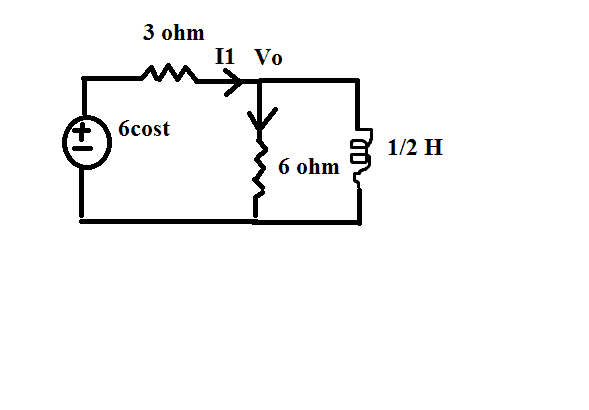# The average power consumed -- where is my mistake

## Homework Statement

We have the circuit in the figure.I have to find the average power consumed by all the elements and the source.P=0.5*(I^2)*R
P=-VmImcosθ/2

## The Attempt at a Solution

The inductor consumes zero inductive power.
I apply nodal analysis at node Vo

We have (6-Vo)/3=Vo/6
Vo=4
Here I find that I1=(6-4)/3=2/3
So the average power dissipated by 3 ohm
P=0.5*(4/9)*3=2/3 W
Current through 6 ohm is Vo/6=4/6
P=0.5*(16/36)*6=4/3..problem is ,in my book power dissipated by 6 ohm is 10/3....
Now,the power consumed by the source is
P=P=-(2/3)(6)cos0/2=-2 W...but the result in my book is -4W
Where is my mistake?

BvU
Homework Helper
How do you calculate ##\theta = 0## if there is an L in the circuit ?

gneill
Mentor
Your book's result of -4 W for the average power "consumed" by the source looks very mysterious to me. I think the source should be delivering more than 4 W to the load.

Assuming that the source voltage function 6cos(t) implies a 6V peak value, then the RMS value would be 6/√2, and the frequency of the source would be ω = 1 rad/sec. Taking the equivalent impedance Z of the load and using the RMS value of the source voltage I find a real power in the load of closer to 6 W. (I say "closer to" because I'm not going to give away actual results here).

BvU
Homework Helper
Here is a cheat sheet. Look here for your load impedance (its complex). I needed it to dust off what I used to know. Didn't end up with the book answer either

BvU